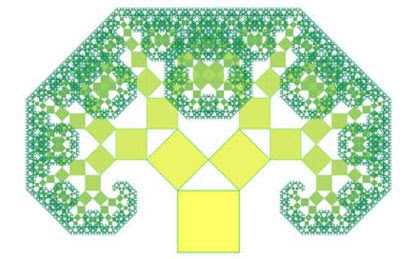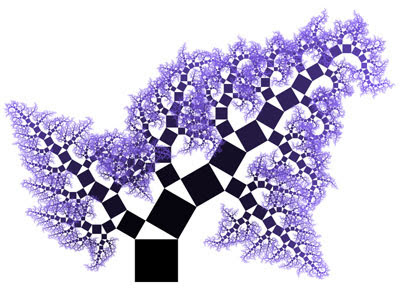# Let’s Plant Pythagoras Trees!

Pythagorean Theorem is one of the most famous theorems in mathematics. Centuries ago many ancient civilizations discovered that the square of a right-angled triangle hypotenuse was equal to the sum of the squares of the other sides, then Pythagoras (c. 570-495 BC) was credited for its first recorded proof.

However, do you know that aside from helping us with right-angled triangle, Pythagorean Theorem can produce a really beautiful thing as well? Take a look at the following Pythagoras tree.Pythagoras tree is a type of fractal which is constructed from squares. In order to make one, we start with one coloured square, at the top of it we construct a right-angled triangle (the angles of the legs can be of any size) such a way that its hypotenuse stick to the side of the square. Then, we build other two coloured squares along the other two sides of the triangle.

Repeat the construction recursively on the other two squares, for as many iterations as you can. The result is what we call as Pythagoras tree.

We can also construct Pythagoras trees with isosceles right triangles as shown in the figure below.Can you guess how the following fractal is made?Finally, what is so special with today’s Pythagoras article? Indeed, 15 August 2017 or 8-15-17, which is a set of Pythagorean triple, is Pythagorean Theorem Day! The next similar days will be on 16 December 2020 and 24 July 2025. How lucky we are to have those days.

Now, it’s time to challenge yourselves to make your own Pythagoras trees.

Source:

http://mathpaint.blogspot.co.id/

https://alexvieth.wordpress.com/

http://mathforum.org/mathimages/index.php/Pythagorean_Tree